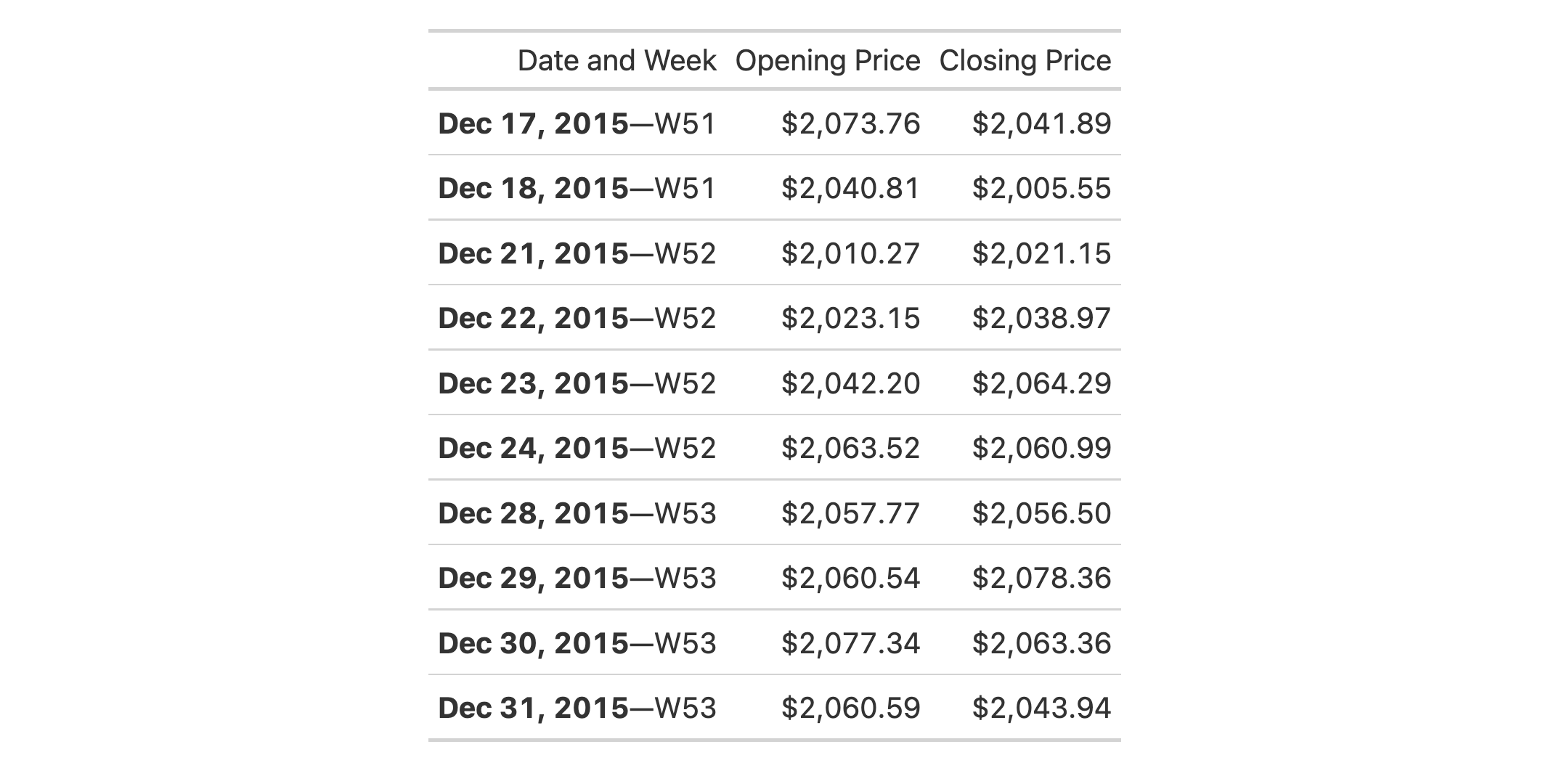# text_transform: Perform targeted text transformation with a function In gt: Easily Create Presentation-Ready Display Tables

 text_transform R Documentation

## Perform targeted text transformation with a function

### Description

Perform targeted text transformation with a function

### Usage

```text_transform(data, locations, fn)
```

### Arguments

 `data` A table object that is created using the `gt()` function. `locations` The cell or set of cells to be associated with the text transformation. Only the `cells_body()`, `cells_stub()`, `cells_column_labels()`, and `cells_row_groups()` helper functions can be used here. We can enclose several of these calls within a `list()` if we wish to make the transformation happen at different locations. `fn` The function to use for text transformation.

### Value

An object of class `gt_tbl`.

### Examples

Use `exibble` to create a gt table. transform the formatted text in the `num` column using a function supplied to `text_transform()` (via the `fn` argument). Note that the `x` in the `fn = function (x)` part is a formatted vector of column values from the `num` column.

```exibble %>%
dplyr::select(num, char, currency) %>%
dplyr::slice(1:4) %>%
gt() %>%
fmt_number(columns = num) %>%
fmt_currency(columns = currency) %>%
text_transform(
locations = cells_body(columns = num),
fn = function(x) {
paste0(
x, " (",
dplyr::case_when(
x > 20   ~ "large",
x <= 20  ~ "small"),
")")
}
)
```### Function ID

3-22

Other data formatting functions: `data_color()`, `fmt_bytes()`, `fmt_currency()`, `fmt_datetime()`, `fmt_date()`, `fmt_duration()`, `fmt_engineering()`, `fmt_fraction()`, `fmt_integer()`, `fmt_markdown()`, `fmt_number()`, `fmt_partsper()`, `fmt_passthrough()`, `fmt_percent()`, `fmt_roman()`, `fmt_scientific()`, `fmt_time()`, `fmt()`, `sub_large_vals()`, `sub_missing()`, `sub_small_vals()`, `sub_values()`, `sub_zero()`